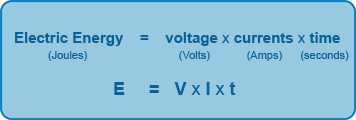## Electrical Energy

This is the energy that results from the flow of electrons.

The energy in an electrical circuit can be calculated from the following formula:### Example

A 230 Volt mains supply is connected to a 3 Amp electric fan for 5 minutes. Calculated the electrical energy?

Solution:

E = V x I x t

E = 230 x 3 x 300 [remember 5 minutes = 5 x 60 = 300 seconds]

E = 207000 Joules = 207 kilojoules (kJ)